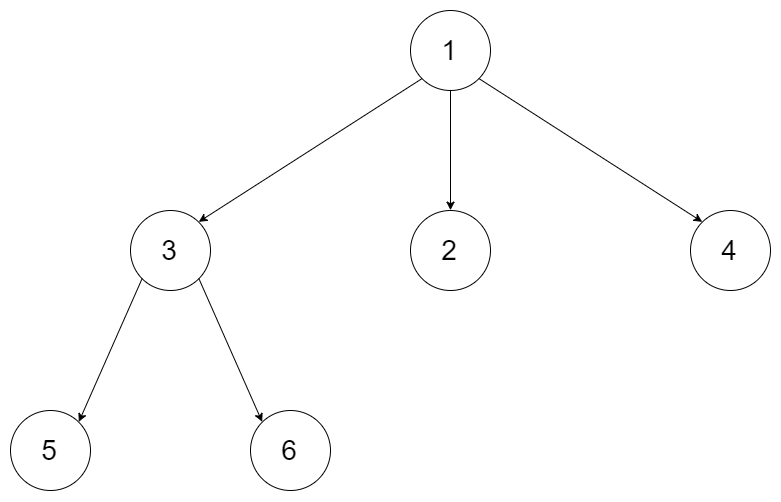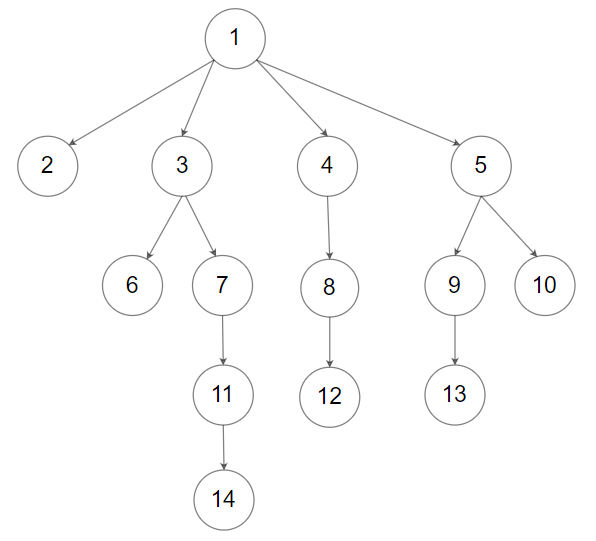# 589. N-ary Tree Preorder Traversal

Easy
Given the `root` of an n-ary tree, return the preorder traversal of its nodes' values.
Nary-Tree input serialization is represented in their level order traversal. Each group of children is separated by the null value (See examples)
Example 1:Input: root = [1,null,3,2,4,null,5,6]
Output:
[1,3,5,6,2,4]
Example 2:Input: root = [1,null,2,3,4,5,null,null,6,7,null,8,null,9,10,null,null,11,null,12,null,13,null,null,14]
Output:
[1,2,3,6,7,11,14,4,8,12,5,9,13,10]
Constraints:
• The number of nodes in the tree is in the range `[0, 104]`.
• `0 <= Node.val <= 104`
• The height of the n-ary tree is less than or equal to `1000`.
Follow up: Recursive solution is trivial, could you do it iteratively?

### 解題

Recursive
/**
* Definition for a Node.
* type Node struct {
* Val int
* Children []*Node
* }
*/
func preorder(root *Node) []int {
res := make([]int, 0)
dfs(root, &res)
return res
}
func dfs(root *Node, arr *[]int) {
if root == nil {
return
}
*arr = append(*arr, root.Val)
for _, n := range root.Children {
dfs(n, arr)
}
}
Iterative
/**
* Definition for a Node.
* type Node struct {
* Val int
* Children []*Node
* }
*/
func preorder(root *Node) []int {
stack := make([]*Node, 0)
res := make([]int, 0)
if root == nil {
return res
} else {
stack = append(stack, root)
}
for len(stack) != 0 {
node := stack[len(stack) - 1]
stack = stack[:len(stack) - 1]
res = append(res, node.Val)
for i:=len(node.Children) - 1; i >= 0; i-- {
stack = append(stack, node.Children[i])
}
}
return res
}